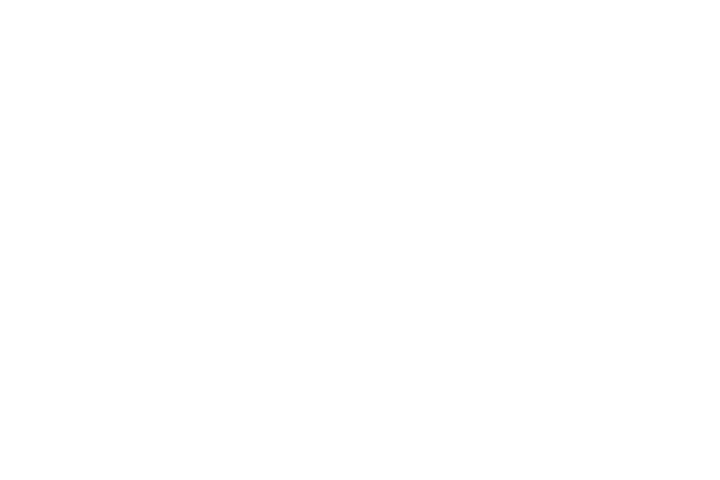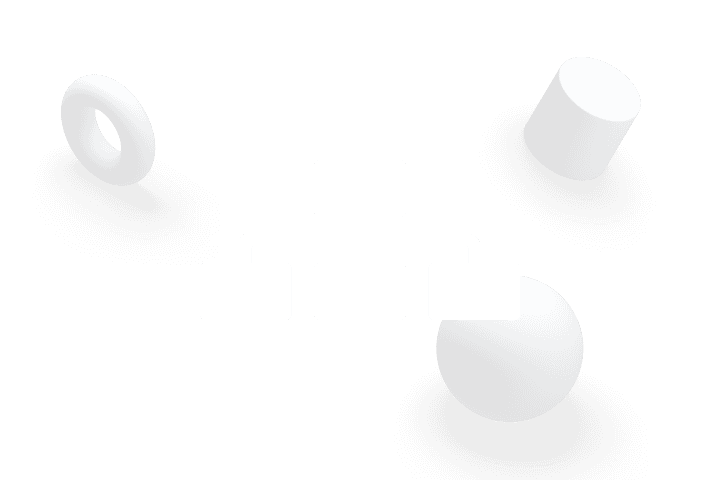Introduction to Data Structures in C++

You will learn the principles of DSA by studying examples from the real world. This course is intended to provide you the chance to think like a real YouTube engineer, developing features and solving issues by making the use of DSA.

Earn a certificate of completion

1-2 months### Course outcomes

Acquire a thorough understanding of all of the popular Data Structures and algorithms

Improve your analytical and problem-solving abilities by studying examples and practicing questions from the real world

Learn to write clear and efficient code by completing practise exercises and tests

### Pre-requisites

Basic understanding of programming and familiar with syntax of C++

### Syllabus

Introduction

Introduction to different data structures, their properties and applications

Algorithm Analysis

Finding time and space complexity of algorithms, learning about ‘Big Oh’ notations

Arrays

Learning the concepts of array indexing, static and dynamic arrays, array traversal and operations on arrays

String

Understanding the properties of string, operations on string, substring and concatenation functions

Searching

Learning about searching techniques, linear and binary search and their time complexities

Recursion

Introduction to recursion, principle of mathematical induction, Fibonacci numbers, recursion using arrays, recursion using strings, list of common problems solved using recursion

Sorting

Introduction to sorting algorithms like selection sort, insertion sort, bubble sort, merge sort, quick sort.

Multidimensional Array

Deeper dive in arrays to learn about 2-D arrays, traversals and programming problems based on them

Introduction to pointers

Learn about pointers in C++, referencing and dereferencing techniques, types of pointers and pointer arithmetic

Implementation of doubly linked list, node insertion and deletion from particular places

Implementation of circular linked list, node insertion and deletion from particular places along with learning cycle finding algorithms

Abstract Data Types

Stacks

Introduction to stacks, properties and applications of stacks, stack using arrays, stack using linked list, Inbuilt stack

Queues

Queue using arrays, properties and applications of queues, Queue using linked list, Inbuilt queue

Hash Maps

Introduction to Hash Maps, Inbuilt Hash Map, Hash functions, Insert and Delete operations, implementation using linked lists and arrays

### Course outcomes

Acquire a thorough understanding of all of the popular Data Structures and algorithms

Improve your analytical and problem-solving abilities by studying examples and practicing questions from the real world

Learn to write clear and efficient code by completing practise exercises and tests

### Pre-requisites

Basic understanding of programming and familiar with syntax of C++

### Syllabus

Introduction

Introduction to different data structures, their properties and applications

Algorithm Analysis

Finding time and space complexity of algorithms, learning about ‘Big Oh’ notations

Arrays

Learning the concepts of array indexing, static and dynamic arrays, array traversal and operations on arrays

String

Understanding the properties of string, operations on string, substring and concatenation functions

Searching

Learning about searching techniques, linear and binary search and their time complexities

Recursion

Introduction to recursion, principle of mathematical induction, Fibonacci numbers, recursion using arrays, recursion using strings, list of common problems solved using recursion

Sorting

Introduction to sorting algorithms like selection sort, insertion sort, bubble sort, merge sort, quick sort.

Multidimensional Array

Deeper dive in arrays to learn about 2-D arrays, traversals and programming problems based on them

Introduction to pointers

Learn about pointers in C++, referencing and dereferencing techniques, types of pointers and pointer arithmetic

Implementation of doubly linked list, node insertion and deletion from particular places

Implementation of circular linked list, node insertion and deletion from particular places along with learning cycle finding algorithms

Abstract Data Types

Stacks

Introduction to stacks, properties and applications of stacks, stack using arrays, stack using linked list, Inbuilt stack

Queues

Queue using arrays, properties and applications of queues, Queue using linked list, Inbuilt queue

Hash Maps

Introduction to Hash Maps, Inbuilt Hash Map, Hash functions, Insert and Delete operations, implementation using linked lists and arrays

### Course outcomes

Acquire a thorough understanding of all of the popular Data Structures and algorithms

Improve your analytical and problem-solving abilities by studying examples and practicing questions from the real world

Learn to write clear and efficient code by completing practise exercises and tests

### Pre-requisites

Basic understanding of programming and familiar with syntax of C++

### Syllabus

Introduction

Introduction to different data structures, their properties and applications

Algorithm Analysis

Finding time and space complexity of algorithms, learning about ‘Big Oh’ notations…

See full syllabus

Standard

₹499/mo

Pay what you want

for the first month

Offer available for a limited time

You can cancel the subscription within the first 7 days of purchase. Read our policy

Standard

₹499/mo

Pay what you want

for the first month

Offer available for a limited time

You can cancel the subscription within the first 7 days of purchase. Read our policy

301/302, 3rd Floor, Saket Callipolis, Sarjapur Main Rd, Doddakannelli, Bengaluru, Karnataka 560035

For any queries, reach out to us at hello@byteavenue.org

For any queries, reach out to us at hello@byteavenue.org

301/302, 3rd Floor, Saket Callipolis, Sarjapur Main Rd, Doddakannelli, Bengaluru, Karnataka 560035